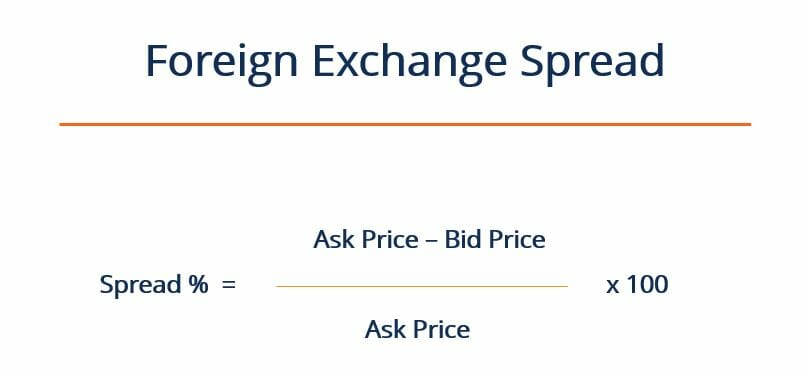# How can We Calculate the Foreign Exchange Spread?What is the Foreign Exchange Spread?
The foreign exchange spread (or bid-ask spread) refers to the difference in the bid and ask prices for a given currency pair. The bid price refers to the maximum amount that a foreign exchange trader is willing to pay to buy a certain currency, and the ask price is the minimum price that a currency dealer is willing to accept for the currency.

How can We Calculate the Foreign Exchange Spread?
The foreign exchange spread is usually expressed as a percentage, and can be calculated using the formula below:Source : CFI

Where:

Ask Price – Refers to the lowest price that a currency dealer is willing to sell units of the currency for

Bid Price – Refers to the highest price that a currency trader is willing to buy units of the currency for

Factors that Influence the Foreign Exchange Spread
There are a great number of factors that can affect the magnitude of bid-ask spreads that prevail on certain trading floors. For example: